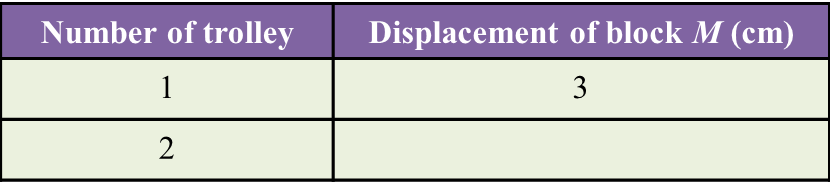# 5.7.1 The Concept of Momentum (Structured Questions)

Question 1:
Diagram 1.1 and Diagram 1.2 show an experiment to study the collision between trolleys of different masses with a plasticine block.Both trolleys move with the same velocity.
(a) State the variables in this experiment.
(i) Manipulated variable [1 mark]
(ii) Responding variable [1 mark]

(b)(i) What can you observe about the plasticine block after the collision? [1 mark]
(ii) State one inference that can be made based on the observation in (b)(i). [1 mark]

(c) State the hypothesis of this experiment. [1 mark]

(a)(i)
Mass of the trolley

(a)(ii)
Depth of the dent on the plasticine block

(b)(i)

Trolley T produced a deeper dent on the plasticine block compared to the dent produced by trolley S after their collision with the plasticine block.

(b)(ii)

When a moving trolley with a bigger mass collides with a plasticine block, it produces a deeper dent on the plasticine block.

(c)
The bigger the mass of a moving trolley which collides with a plasticine block, the deeper the dent which will be formed on the plasticine block.

Question 2:
Diagram 2.1 shows an experiment to study the collision of a trolley with block M. After collision, block M is displaced to a new position.
The experiment is repeated using two trolleys as shown in Diagram 2.2.The result of this experiment is shown in Table below.(a) Measure the displacement of block M after the collision in Diagram 2.2.  [1 mark]

(b) State one observation about displacement of block M in diagram 2.1 and 2.2[1 mark]

(c) State the variables in this experiment.
(i) Constant variable [1 mark]
(ii) Manipulated variable [1 mark]

(d) Predict the displacement of block M if three trolleys are used. [1 mark]

(a) 6 cm

(b)
The displacement of block M increases when the number of trolley increases.

(c)(i)
Length and slope of runway, mass of block M.

(c)(ii)
The number of trolley used

(d)
9 cm

# 5.1 Indices

5.1 Indices

5.1.1 Indices
1.   A number expressed in the form an is known as an index notation.
2.   an is read as ‘a to the power of n’ where a is the base and n is the index.
Example:

3.
If a is a real number and is a positive integer, then

4.   The value of a real number in index notation can be found by repeated multiplication.
Example:
6= 6 × 6 × 6 × 6
= 1296

5.
A number can be expressed in index notation by dividing the number repeatedly by the base.
Example:
243 = 3 × 3 × 3 × 3 × 3

5.1.2 Multiplication of Numbers in Index Notation
The multiplication of numbers or algebraic terms with the same base can be done by using the Law of Indices.

am  × an = am + n

Example:
33 × 38 = 33+8
= 311

5.1.3 Division of Numbers in Index Notation
1. The Law of indices for division is:

am  ÷ an = am - n

Example:
412 ÷ 412 = 412-12
= 40
= 1

2. a0 = 1

5.1.4 Raise Numbers and Algebraic Terms in Index Notation to a Power
To raise a number in index notation to a power, multiply the two indices while keeping the base unchanged.

(am ) n  = (an ) m  = amn

Example:
(43)7 = 43×7
= 421

5.1.5 Negative Indices

${a}^{-n}=\frac{1}{{a}^{n}}$

Example:
${5}^{-2}=\frac{1}{{5}^{2}}=\frac{1}{25}$

5.1.6 Fractional Indices

Example: